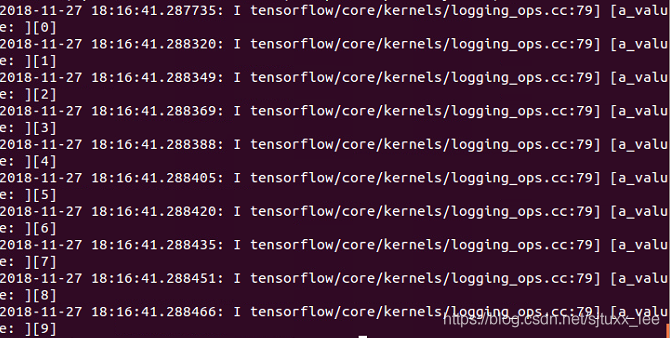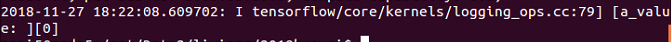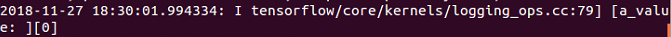# Tensorflow in function tf.Print Method of outputting intermediate value

tensorflow because of its model based on the static map, lead to writing the code is hard to debug, besides using official debugging tools, the most direct way is to put the intermediate result output out of view, however, use the print function can only output directly the shape of a tensor variable, rather than numerical, want to use specific numerical output tensor needs tf. The print function. There are many instructions on the web about how to use this function. Here is a brief description:

``````Print(
input_,
data,
message=None,
first_n=None,
summarize=None,
name=None
)
``````

parameter:

• input_ : tensor that passes through this operation.
• data: list of tensors to print when calculating op.
• message: a string, the prefix for the error message.
• first_n: record first_n times only. Negative log, which is the default.
• : print only a fixed number of entries for each tensor. If not, each input tensor prints up to three elements. Name: name of the operation (optional)

however, most of the resources on the web describe how to set up an op in the main function and then open a Session to execute sess.run(op), but what if you want to output an intermediate value in the function that does not return to the main function?In this case, a new Session cannot be opened in the function, but you can still create an op using TF.print.

``````import tensorflow as tf
import os

os.environ["CUDA_VISIBLE_DEVICES"] = "0"

def test():
a=tf.constant(0)
for i in range(10):
a_print = tf.Print(a,['a_value: ',a])
a=a_print+1
return a

if __name__=='__main__':
with tf.Session() as sess:
sess.run(test())
``````

operation result:a_print can be understood as a new node in the figure. In the following code, when another variable USES a_print (example a=a_print+1), there will be data flowing from a_print node, and the value will be output. But how many times will the value be output?In fact, it is not how many times a_print is used in the following text, but how many times the data flow must flow from this node, which can be interpreted as how many times the OP of A_print is “defined”.

``````def test():
a=tf.constant(0)
a_print = tf.Print(a,['a_value: ',a])
for i in range(10):
a=a_print+1
return a

if __name__=='__main__':
with tf.Session() as sess:
sess.run(test())
``````

if the test () function to this way, the operation result is:output is performed only once, because a_print the op is defined only once, although back in circulation has been a used, but the data from it after only once, so will only print once, and a_print value is 0, always ultimately return a value of 1.
then change the code to the following example:

``````def test():
a=tf.constant(0)
a_print = tf.Print(a,['a_value: ',a])
for i in range(10):
a_print=a_print+1
return a

if __name__=='__main__':
with tf.Session() as sess:
sess.run(test())
``````

The result of running

will not output anything, because the op of a_print is not related to any other variable, it is not used by any other variable, it is an isolated node in the graph, no data flow, it will not be executed.
and if I change this to

``````def test():
a=tf.constant(0)
a_print = tf.Print(a,['a_value: ',a])
for i in range(10):
a_print=a_print+1
return a_print

if __name__=='__main__':
with tf.Session() as sess:
sess.run(test())
``````

run resultreturns an a_print value of 10, which is also correct, because a_print is returned later, so there is a data flow through it and it will be executed, while a_print is only executed once because the definition of a_print is only executed once.